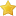Themabewertung:
• 0 Bewertung(en) - 0 im Durchschnitt
• 1
• 2
• 3
• 4
• 5
 Question about a solving techniqueSirWoezelFillominolöserBeiträge: 4 Themen: 1 Registriert seit: Jun 2020 Bewertung: 0 09.06.2020, 14:50 Hi all, I am working on a puzzle (sudoku) which ends in a situation where all cells are filled in except these:  x  x  x  ab x  abc ac  x  x  x  x  x  x  x  ac  ac  x  x  x  x  x  x  x  x   x   x  x  x  x  x  x  x  x   x   x  x  x  x  x  x  x  x   x   x  x  x  x  x  x  x  x   x   x  x  x  x  x  x  x  x   x   x  x  x  x  x  ab x  ab  x   x  x  x  x  x  x  x  x   x   x  x The X's are not important. Only the 7 cells with candidates abc in them are. As I have blundered with this before, I thought I'd just ask here: is this an ok BUG that allows the solver to deduct that R2C6 cannot be a c (because otherwise you would end up with two solutions)? Thanks in advance, SirWoezel